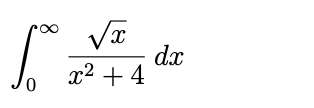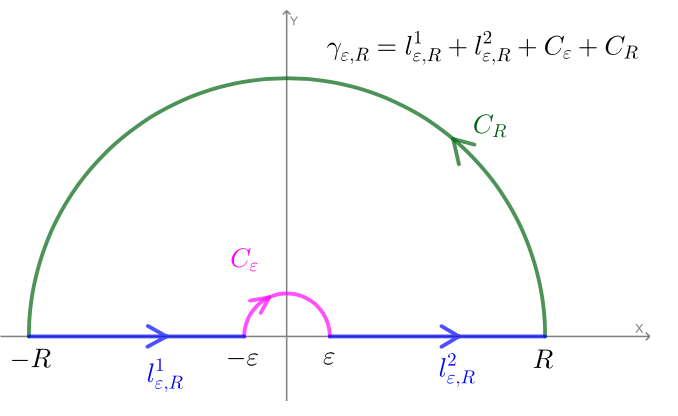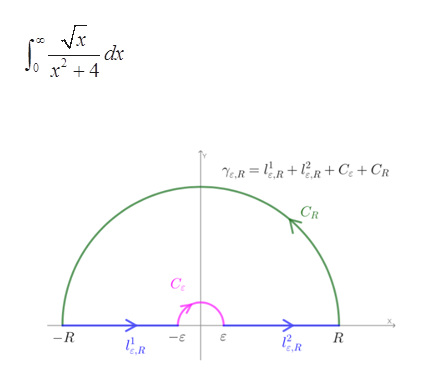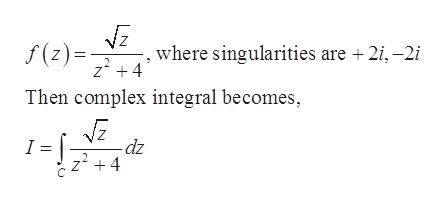Question
130 views

Use complex contour integration to evaluate the following integrals:

Use an appropriate branch of log z so you can use the following contour.help_outlineImage Transcriptionclosedx x2 +4 fullscreenhelp_outlineImage Transcriptionclose12 ‚R Ye,R = lR+ V%R+ C; + CR ‚R CR C: -R R -E ‚R fullscreen
check_circle

Step 1

Given integral and contour are,help_outlineImage Transcriptionclosedx x + 4 Ye,R = 1R+R+C; + Cr CR Ce R -R c.R fullscreen
Step 2

To evaluate the given integration, the complex-values function is,help_outlineImage Transcriptionclosef(z) =: z' +4 where singularities are + 2i, –2i Then complex integral becomes, -dz z' +4 fullscreen
Step 3

It can be solved by using residue theorem or Cauchy integral formula. Here z​1⁄2 = e​1⁄2Log z,

then z​1⁄2 has a branch cut. The logarithm branch cut is defined as the negative real axis. So, define it to be the positive real axis. Hence, use keyhole contour, which consists of a small circle about the origin of radius ε say, extending to a line seg...

### Want to see the full answer?

See Solution

#### Want to see this answer and more?

Solutions are written by subject experts who are available 24/7. Questions are typically answered within 1 hour.*

See Solution
*Response times may vary by subject and question.
Tagged in

### Math